# Specify Fixed-Point Math Properties in MATLAB Function Block

This example shows how to specify fixed-point math properties in a MATLAB® Function block. You can specify `fimath` properties to add fixed-point numbers without bit growth. The same methods also apply to subtraction and multiplication.

### Define Fixed-Point Variables with Attached `fimath`

Define fixed-point variables `A` and `B` with attached `fimath`.

```clearvars F = fimath(... 'RoundMode','Fix',... 'OverflowMode','Wrap',... 'ProductMode','SpecifyPrecision',... 'ProductWordLength',32,... 'ProductFractionLength',16,... 'SumMode','SpecifyPrecision',... 'SumWordLength',32,... 'SumFractionLength',16,... 'CastBeforeSum',true); A = fi(1,true,32,16,F); B = fi(1,true,32,16,F);```

Define a structure `T` containing prototypes of the variables `A` and `B`.

```T.A = cast(0,'like',A); T.B = cast(0,'like',B);```

The structure `T` functions as a types table. The values of the fields of `T` are not important. You will use the data types of the fields of `T` later in this example to specify fixed-point types that carry the `fimath` along with them.

Add the fixed-point variables `A` and `B`. In MATLAB, the `fimath` attached to variables `A` and `B` specify that the sum is to be computed as 32-bit word length and 16-bit fraction length.

`Y = A + B`
```Y = 2 DataTypeMode: Fixed-point: binary point scaling Signedness: Signed WordLength: 32 FractionLength: 16 RoundingMethod: Zero OverflowAction: Wrap ProductMode: SpecifyPrecision ProductWordLength: 32 ProductFractionLength: 16 SumMode: SpecifyPrecision SumWordLength: 32 SumFractionLength: 16 CastBeforeSum: true ```

The `fimath` propagates to the variable `Y`.

### Default MATLAB Function Block Behavior

In general, the Simulink software does not propagate `fimath` on fixed-point `fi` objects. This rule applies even if the `fi` objects have attached `fimath` in a Constant block or are passed in as a MATLAB Function block parameter. However, any attached `fimath` defined inside a MATLAB Function block are respected. One exception to the rule about parameters is described in the next section.

This function is defined in the block named `MATLAB Function 1` in the `mParameterFIMath` model. If you execute this function in MATLAB, it returns the same 32-bit data type as the `Y = A + B` example.

```function Y1 = default_behavior(A,B) Y1 = A + B; end ```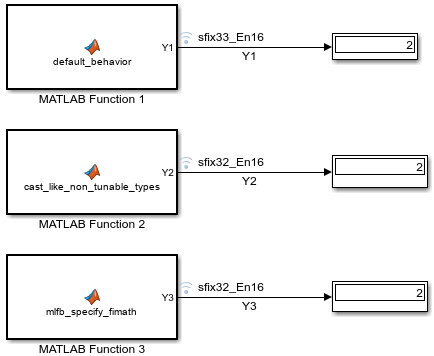Simulate the `mParameterFIMath` model to see how the MATLAB Function block executes this code.

```model = 'mParameterFIMath'; open_system(model); sim(model); Y1 = logsout.get('Y1').Values.Data ```
```Y1 = 2 DataTypeMode: Fixed-point: binary point scaling Signedness: Signed WordLength: 33 FractionLength: 16 ```

The `MATLAB Function 1` block returns `Y1` with a 33-bit word length instead of the 32-bit word length returned in MATLAB. To see why, open the Property Inspector pane for the `MATLAB Funtion 1` block.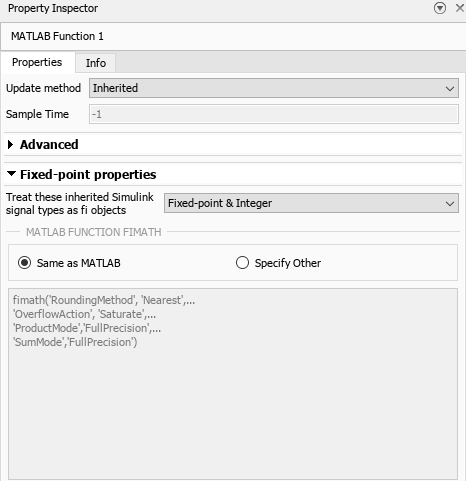The `MATLAB Function 1` block returns a 33-bit word length output because its MATLAB Function `fimath` setting has been set to Same as MATLAB. The input parameters `A` and `B` are stripped of their attached `fimath` and instead use the default `fimath` settings from MATLAB. The default `fimath` in MATLAB does full-precision sums. Therefore, the sum of two 32-bit variables return a 33-bit result.

To see the default `fimath` settings in MATLAB, first reset any global `fimath` settings, then enter `fimath` in the MATLAB Command Window.

```resetglobalfimath fimath```
```ans = RoundingMethod: Nearest OverflowAction: Saturate ProductMode: FullPrecision SumMode: FullPrecision ```

You can also to change the value of `globalfimath`, but doing so changes the fixed-point math behavior globally. This method is not recommended.

### Pass `fimath` into MATLAB Function Block from Parameter

In general, the MATLAB Function block strips `fimath` from fixed-point inputs. The exception to this rule is if an input parameter is a non-tunable structure, such as this one.

```T.A = cast(0,'like',A); T.B = cast(0,'like',B); ```

In the `mParameterFIMath` model, the `MATLAB Function 2` block has the structure `T` defined as a non-tunable input parameter.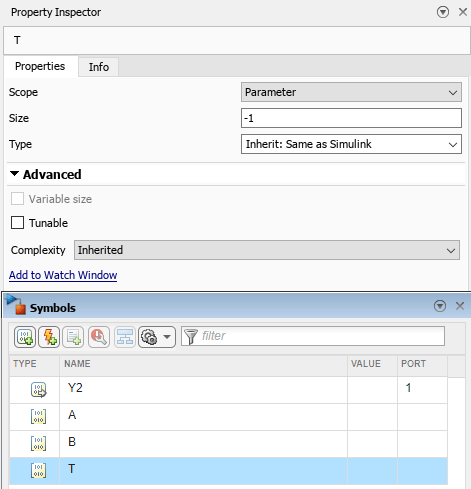The fields `T.A` and `T.B` carry the data type and `fimath` of `A` and `B`. If you cast the inputs `A` and `B` like `T.A` and `T.B`, respectively, you recover the `fimath` that was defined in MATLAB.

```function Y2 = cast_like_non_tunable_types(A, B, T) A = cast(A,'like',T.A); B = cast(B,'like',T.B); Y2 = A + B; end ```

The output of the `MATLAB Function 2` block has the desired 32-bit word length.

`Y2 = logsout.get('Y2').Values.Data`
```Y2 = 2 DataTypeMode: Fixed-point: binary point scaling Signedness: Signed WordLength: 32 FractionLength: 16 ```

This workflow provides a robust way of defining fixed-point data types in MATLAB Function blocks because it allows for the definition of different `fimath` and data type for each different variable. This method has these advantages:

• The algorithm and type specification can be separate, with the types controlled in a dictionary separate from the block. Changing the type does not change the algorithm or block.

• MATLAB and MATLAB Function blocks in Simulink have identical behavior.

• Each parameter can have its own `fimath` and data type.

• Each parameter can change to be types other than fixed-point. For instance, `T.A = single(0); T.B = single(0);` would change all types in this example to single without having to use global data-type override settings.

### Set `fimath` for All Input Parameters

An alternative way to define `fimath` in a MATLAB Function block is to declare the `fimath` in the Fixed-point properties of the MATLAB Function block.

In the `mParameterFIMath` model, the `MATLAB Function 3` block contains this code.

```function Y3 = mlfb_specify_fimath(A, B) Y3 = A + B; end ```

In the Property Inspector pane, under Fixed-point properties > MATLAB Function `fimath`, the option Specify Other is selected. The `fimath` is defined as the variable `F` from above.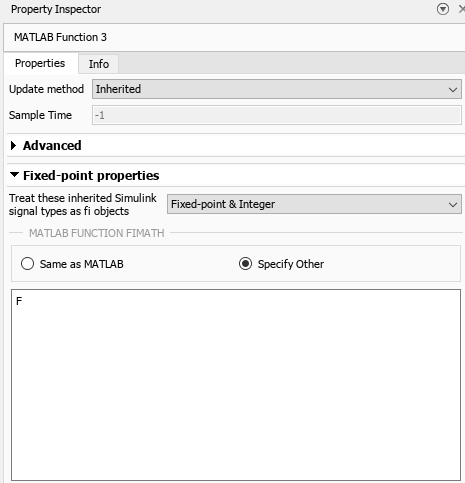Alternatively, you can write the `fimath` definition directly in the MATLAB Function `fimath` box.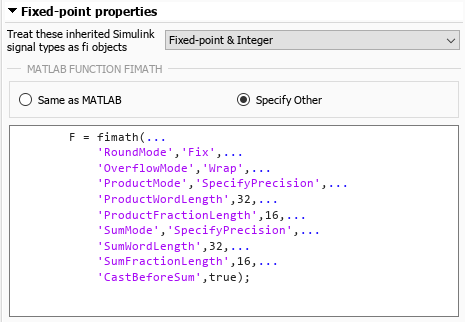Confirm that the output of this block has the desired 32-bit word length.

`Y3 = logsout.get('Y3').Values.Data `
```Y3 = 2 DataTypeMode: Fixed-point: binary point scaling Signedness: Signed WordLength: 32 FractionLength: 16 ```

This method has these limitations:

• All MATLAB Function block input parameters get the same `fimath`.

• Each MATLAB Function block must be modified to specify the `fimath`.